SYLLABUS  Previous: 3.4 Implementation and solution  Up: 3 FINITE ELEMENT METHOD  Next: 3.6 Variational inequalities

## 3.5 Linear solvers

Slide : [ Direct LU || Iterative splitting : Jacobi - Gauss-Seidel - SOR - Preconditioning || VIDEO login]

Writing efficient solvers for linear systems is a complete chapter of numerical analysis and involves much more than what can be said in this section. As a user of software libraries such as Netlib  or PetSc , it is however sufficient to have only a rough idea of what type of solvers exist and how to used them.Direct LU factorization.
Remember that you should a never calculate a matrix inverse to solve a linear system; the solution can be calculated with only one third of the number of operations by decomposing the matrix into Lower and Upper triangular parts [3.9] with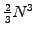arithmetic operations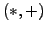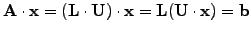(1)

and later use a forward and backward substitution of the right hand side vector involving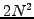operations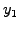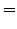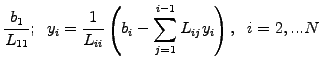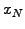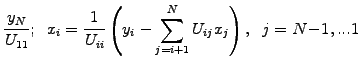(2)

A particularly simple version has been implemented in JBONE for tri-diagonal matrices, where the solve3() method computes the solution directly in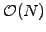operations; the first and the last equations are simply eliminated by hand'' to take care of the periodicity. Many different implementations of one and the same LU-factorization exist taking advantage of the specific pattern of the matrix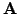; before you start writing your own, check Netlib and it is likely that you will find a routine tailored for your application.

When the matrix has more than three diagonals, it is important to allow for a pivoting during the factorization process - interchanging rows and columns in the matrix to avoid divisions by small numbers on the diagonal. For particularly large problems, note the possibility of storing the matrix on disk in a frontal implementation of the same algorithm.

When memory consumption, calculation time or parallelization become an issue in 2, 3 or even higher dimensions, it might be useful to consider iterative solvers as an alternative to the direct LU decomposition. The idea behind them is best understood from a splitting of the matrix into a sum17of a diagonal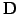, strict lower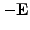and upper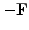triangular parts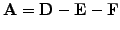(3)

An iterative solution of the problem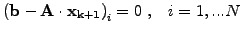(4)

is then sought where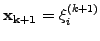is the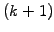-th approximation of the solution having components from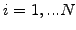.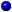Iterative Jacobi.
The simplest form of iteration consists in inverting only the diagonal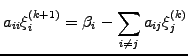(5)

which is equivalent in matrix notation to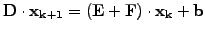(6)

Starting from an arbitrary initial guess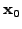, simple elliptic problems will -albeit slowly- converge to a stationary point which is the solution of the linear problem.Gauss-Seidel.
As the equationsare updated one after the other, it is possible to immediately use the updated components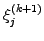using forward Gauss-Seidel iterations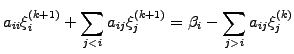(7)

or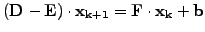(8)

where the calculation is carried out with the equation index increasing from. The reverse is also possible and is called backward Gauss-Seidel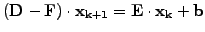(9)Successive over-relaxations - SOR
are obtained when one of the Gauss-Seidel iterations (3.5#eq.8 or 3.5#eq.9) is linearly combined with the value of the previous step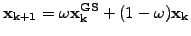(10)

using the parameter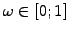for an under-relaxation or rather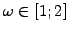for an over-relaxation. A symmetric-SOR (SSOR) scheme is obtained with a combination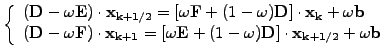(11)Preconditioning / approximate inverse.
Realizing that all the methods above are of the form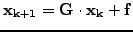where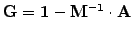, the linear problem can be diagonalized approximatively using a preconditioning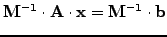(12)

where the preconditioner matrix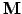is one of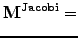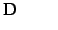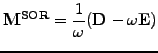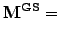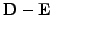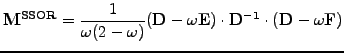The product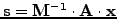is calculated without ever forming the inverse, calculating first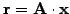and then solving the linear system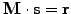.

All these variants of Gauss-Seidel are simple and work fairly well for reasonable sized (e.g.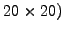elliptic problems - making the much more complicated multigrid approach attractive only for large problems that have to be solved many times. Hyperbolic (wave-) problems and non-Hermitian operators are more difficult to solve iteratively and remains a matter of research ,. Efficient methods in general rely on a suitable preconditioning and the rapid convergence of Krylov or Lanczos methods, such as the generalized minimal residuals (GMRES) and the quasi-minimal residuals (QMR) [3.9].

Note that the matrix-vector multiplications that are used to generate new iterates can be performed in sparse format, i.e. using only those matrix elements that are different from zero. This is the reason why iterative methods can potentially be much more economical than direct solvers - if they converge.

SYLLABUS  Previous: 3.4 Implementation and solution  Up: 3 FINITE ELEMENT METHOD  Next: 3.6 Variational inequalities